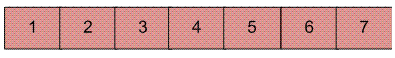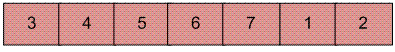GeeksforGeeks App
Open AppBrowser
Continue

# Python Program for Reversal algorithm for array rotation

Write a function rotate(arr[], d, n) that rotates arr[] of size n by d elements.

Example :

```Input :  arr[] = [1, 2, 3, 4, 5, 6, 7]
d = 2
Output : arr[] = [3, 4, 5, 6, 7, 1, 2]
```Rotation of the above array by 2 will make array## Recommended: Please solve it on “PRACTICE ” first, before moving on to the solution.

 `# Python program for reversal algorithm of array rotation` `# Function to reverse arr[] from index start to end``def` `rverseArray(arr, start, end):``    ``while` `(start < end):``        ``temp ``=` `arr[start]``        ``arr[start] ``=` `arr[end]``        ``arr[end] ``=` `temp``        ``start ``+``=` `1``        ``end ``=` `end``-``1` `# Function to left rotate arr[] of size n by d``def` `leftRotate(arr, d):``    ``n ``=` `len``(arr)``    ``rverseArray(arr, ``0``, d``-``1``)``    ``rverseArray(arr, d, n``-``1``)``    ``rverseArray(arr, ``0``, n``-``1``)` `# Function to print an array``def` `printArray(arr):``    ``for` `i ``in` `range``(``0``, ``len``(arr)):``        ``print` `(arr[i])` `# Driver function to test above functions``arr ``=` `[``1``, ``2``, ``3``, ``4``, ``5``, ``6``, ``7``]``leftRotate(arr, ``2``) ``# Rotate array by 2``printArray(arr)` `# This code is contributed by Devesh Agrawal`

Output :

`3 4 5 6 7 1 2`

Time Complexity : O(n)
Auxiliary Space : O(1)

Please refer complete article on Reversal algorithm for array rotation for more details!

My Personal Notes arrow_drop_up